International
Tables for
Crystallography
Volume G
Definition and exchange of crystallographic data
Edited by S. R. Hall and B. McMahon

International Tables for Crystallography (2006). Vol. G, ch. 3.5, pp. 142-143

## Section 3.5.3.2. Atom-centred multipole expansion functions

P. R. Mallinsona* and I. D. Brownb

aDepartment of Chemistry, University of Glasgow, Glasgow G12 8QQ, Scotland, and bBrockhouse Institute for Materials Research, McMaster University, Hamilton, Ontario, Canada L8S 4M1
Correspondence e-mail:  paul@chem.gla.ac.uk

#### 3.5.3.2. Atom-centred multipole expansion functions

| top | pdf |

Data items in this category are as follows:

ATOM_RHO_MULTIPOLEThe bullet • indicates a category key. The arrowis a reference to a parent data item.

Data items in this category are also looped. The category key ( _atom_rho_multipole_atom_label) is also a child of _atom_site_label in the ATOM_SITE category, thereby linking the electron density multipole coefficients to a specific atom site in the list of atom coordinates. This category contains all the parameters of the Hansen & Coppens (1978) function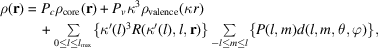where r is a position vector with respect to the atomic nucleus, and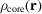and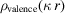are the spherical core and valence electron densities, respectively. They are obtained from atomic orbital analytic wavefunctions such as those tabulated by Clementi & Roetti (1974). They are also the Fourier transforms of the X-ray scattering factors given in _atom_rho_multipole_scat_core and _atom_rho_multipole_scat_valence.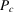is the weight applied to the free-atom core (_atom_rho_multipole_coeff_Pc),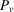is the weight applied to the free-atom valence shell (_atom_rho_multipole_coeff_Pv),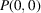is the number of remaining electrons (_atom_rho_multipole_coeff_P00) and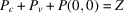(the atomic number) for a neutral atom.is the valence electron expansion factor (_atom_rho_multipole_kappa),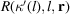is the radial function (Slater or equivalent) (_atom_rho_multipole_radial_*),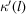is the multipole function expansion factor (_atom_rho_multipole_kappa_prime[l]),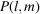are the spherical harmonic coefficients (_atom_rho_multiple_coeff_P[lm]) and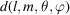is the spherical harmonic of order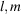at the angle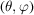. The summations are performed over the index ranges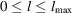,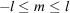, where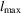is the highest order of multipole applied.

Example 3.5.3.2demonstrates how the category is used in the proton sponge complex of Example 3.5.3.1. Only the first atom is shown in the example.

#### Example 3.5.3.2. Multipole expansion for an atom in the proton sponge complex of Example 3.5.3.1.### References

Clementi, E. & Roetti, C. (1974). Roothan–Hartree–Fock atomic wavefunctions. Basis functions and their coefficients for ground and certain excited states of neutral and ionized atoms. At. Data Nucl. Data Tables, 14, 177–478.Google Scholar
Hansen, N. K. & Coppens, P. (1978). Testing aspherical atom refinements on small-molecule data sets. Acta Cryst. A34, 909–921.Google Scholar